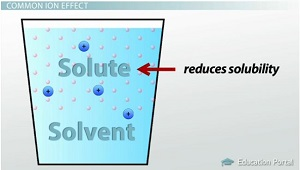# The Common Ion Effect and Selective Precipitation

An error occurred trying to load this video.

Try refreshing the page, or contact customer support.

Coming up next: Acid-Base Equilibrium: Calculating the Ka or Kb of a Solution

### You're on a roll. Keep up the good work!

Replay
Your next lesson will play in 10 seconds
• 0:05 Common Ion Effect
• 2:33 Equilibrium Calculations
• 5:18 Predicting Precipitates
• 6:00 Lesson Summary
Save Save

Want to watch this again later?

Timeline
Autoplay
Autoplay
Speed Speed

#### Recommended Lessons and Courses for You

Lesson Transcript
Instructor: Amy Meyers

Amy holds a Master of Science. She has taught science at the high school and college levels.

Learn what the common ion effect is, how to make equilibrium calculations involving it, and how to find the concentrations of ions when adding reactions in equilibrium to solutions that already contain ions.

## Common Ion Effect

The common ion effect is the phenomenon in which the addition of an ion common to two solutes causes precipitation or reduces ionization. You know Le Chatelier's principle, which states that a reaction stays in equilibrium unless acted on by an outside force; then the reaction will shift to accommodate the force and re-establish equilibrium. This means that if you want a reaction to shift, you just apply an outside force, such as temperature, concentration or pressure. In the case of the common ion effect, a reaction can be shifted by adding an ion that is common to both solutes, thus changing the concentration of the ion in solution and shifting the equilibrium of the reaction. The net effect of the common ion is that it reduces the solubility of the solute in the solution. The common ion effect can make insoluble substances more insoluble.An example of the common ion effect is when sodium chloride (NaCl) is added to a solution of HCl and water. The hydrochloric acid and water are in equilibrium, with the products being H3O+ and Cl- . Then, some sodium chloride is added to the solution.

• HCl + H2O --> H3O+ + Cl-
• NaCl makes Na+ + Cl-

The NaCl dissolves into the solution, forming Na+ and Cl-. As the NaCl dissolves, the concentration of Cl- ions increases. The system accommodates by combining the Na+ and Cl- back into NaCl, which is a solid and precipitates out of the solution. In effect, more of the Cl- ions, which are common to both of the reactions, were added to the solution in equilibrium, so the equilibrium shifted back to the left.

What would happen to the solubility of a saturated solution of MgCO3 if you added K2CO3?

• MgCO3 makes Mg2+ + CO32-
• K2CO3 makes 2K+ + CO32-

So, by adding the K2CO3, the concentration of CO32- ions increases, which causes the reaction equilibrium to shift to the left, which makes more MgCO3, and the solubility decreases.

## Equilibrium Calculations

Let's look at an example. If AgCl is dissolved in a solution that already has a Cl- ion concentration of 0.05 M, what happens to the solubility of AgCl? The Ksp of AgCl is 1.8 * 10^-10. Let's start with the equations:

• AgCl makes Ag+ + Cl-
• Ksp (the solubility constant) = [Ag+] * [Cl-]

Since there are equal moles of Ag+ and Cl- in the solution, we know that their concentration is the same, and we can let x denote their concentration.

For the Cl- concentration, the dissolving of AgCl adds more Cl- to the amount of Cl- already present in the solution, which is 0.05 M. The whole equation becomes 1.8 * 10^-10 = [x] * [x + 0.05].

To unlock this lesson you must be a Study.com Member.

### Register to view this lesson

Are you a student or a teacher?

#### See for yourself why 30 million people use Study.com

##### Become a Study.com member and start learning now.
Back
What teachers are saying about Study.com

### Earning College Credit

Did you know… We have over 200 college courses that prepare you to earn credit by exam that is accepted by over 1,500 colleges and universities. You can test out of the first two years of college and save thousands off your degree. Anyone can earn credit-by-exam regardless of age or education level.